Graphing linear equations By Robert O Mills Jr

• Slides: 21Graphing linear equations By Robert O. Mills Jr. Hermitage High School Henrico County Public SchoolsPart Three GraphingAfter you have discovered a relation of at least 3 ordered pairs you may now graph the linear equation.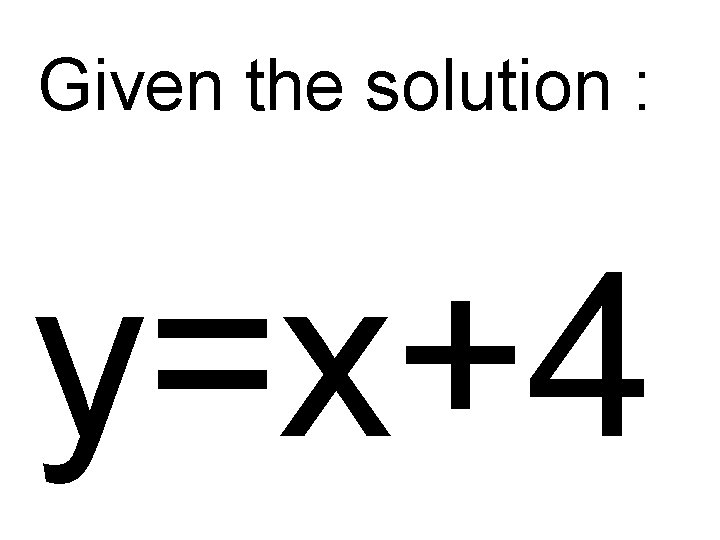Given the solution : y=x+4Create a t-table with the domain (x values) as {-1, 0, 1}. y x -1 3 0 4 1 5Place the points on a graph. x y -1 3 0 4 1 5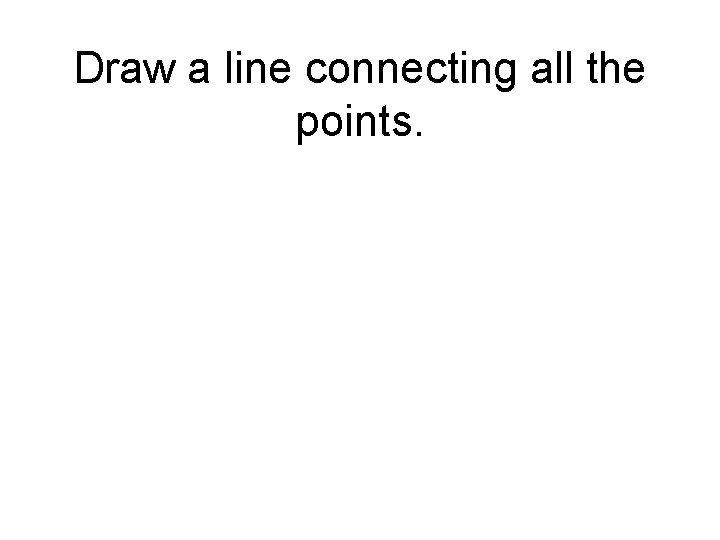Draw a line connecting all the points.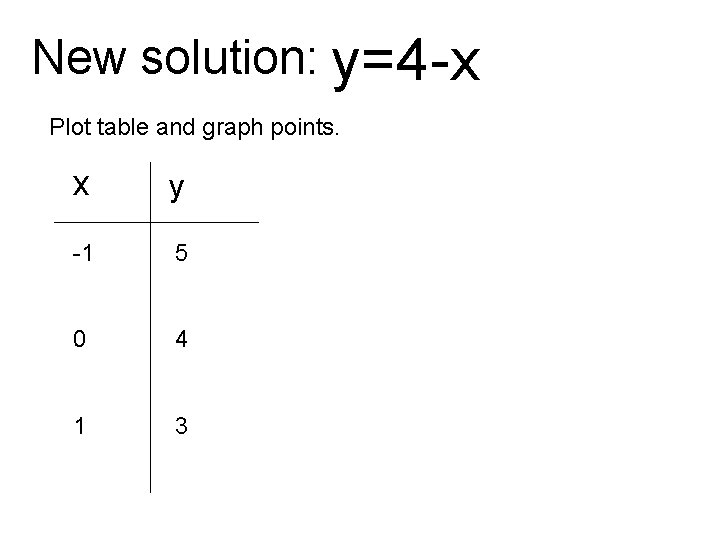New solution: y=4 -x Plot table and graph points. x y -1 5 0 4 1 3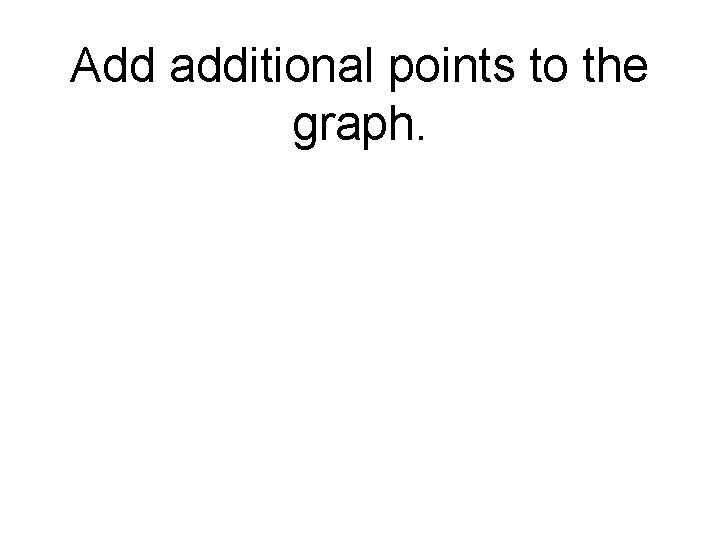Draw a line connecting all the points.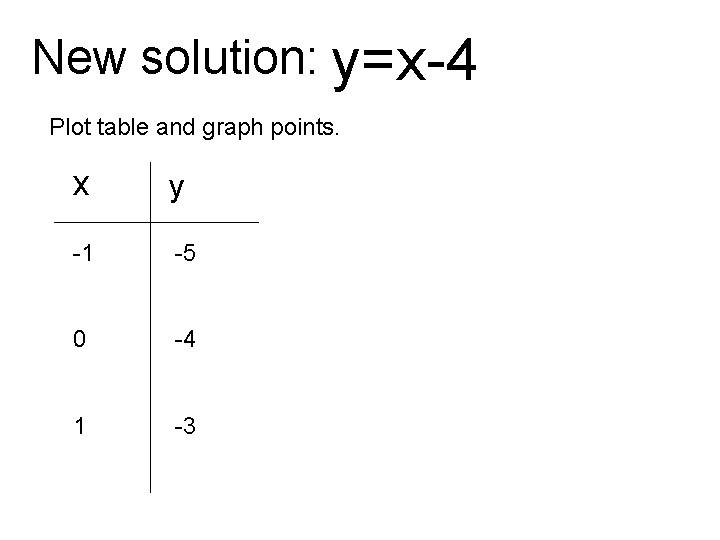New solution: y=x-4 Plot table and graph points. x y -1 -5 0 -4 1 -3Draw a line connecting all the points.New solution: Plot table and graph points. x y -1 2. 5 0 2 1 1. 5Draw a line connecting all the points.New solution: Plot table and graph points. x y -1 -3 0 -2 1 -2